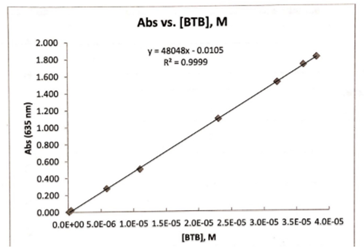# Problem: Use the following graph to determine the molar absorptivity of Bromothymol blue (BTB)? Assume a cuvet with a 14.45 mm diameter was used.

###### FREE Expert Solution
82% (342 ratings)
###### Problem Details

Use the following graph to determine the molar absorptivity of Bromothymol blue (BTB)? Assume a cuvet with a 14.45 mm diameter was used.What scientific concept do you need to know in order to solve this problem?

Our tutors have indicated that to solve this problem you will need to apply the Calculate Molarity concept. You can view video lessons to learn Calculate Molarity. Or if you need more Calculate Molarity practice, you can also practice Calculate Molarity practice problems.

What is the difficulty of this problem?

Our tutors rated the difficulty ofUse the following graph to determine the molar absorptivity ...as high difficulty.

How long does this problem take to solve?

Our expert Chemistry tutor, Dasha took 4 minutes and 21 seconds to solve this problem. You can follow their steps in the video explanation above.

What professor is this problem relevant for?

Based on our data, we think this problem is relevant for Professor Staff's class at UIC.